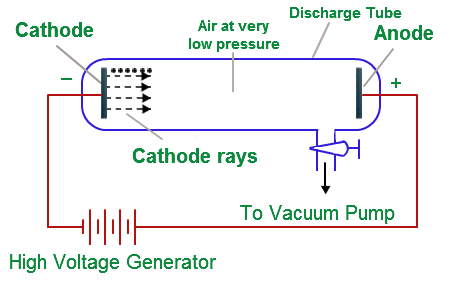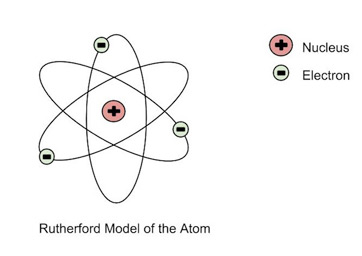# CBSE Class 11 Chemistry Chapter 2 Revision Notes

Chapter 2: Structure of the Atom Revision Notes

Introduction to Atomic Structure

• Atoms are the fundamental components of matter. The proton, neutron, and electron are the three subatomic particles that make up the smallest unit of matter.

Experiment with cathode raysSource:

• The presence of electrons was discovered by J. J. Thomson.
• He accomplished this by employing a cathode ray tube, which is a vacuum-sealed tube with a cathode and anode on one end that produces an electron beam that travels to the other end.
• High voltage is applied to the air inside the chamber, and electricity flows through it from the negative electrode to the positive electrode.
• The material of the electrodes and the type of the gas present in the cathode ray tube have no effect on the properties of cathode rays (electrons).
• The experiment demonstrated that the atom is not a simple, indivisible entity, but rather contains at least one subatomic particle, the electron.

Electrons

• An atom’s electrons are negatively charged subatomic particles.
• An electron’s mass is deemed insignificant, and its charge is -1.
• The electron’s symbol is e–.
• Electrons are very minuscule particles.
• They exist outside of the nucleus.

A model of an atom by Thomson

• An atom, according to Thomson(i), is made up of a positively charged sphere with electrons contained within it. (ii) The magnitudes of the negative and positive charges are equal. As a result, the atom is electrically neutral as a whole.
• The first atomic model to be proposed and taken into consideration.
• He recommended that the atom be modelled after a Christmas pudding or watermelon.
• The positive charge in the atom is compared to the red edible section of the watermelon.
• The watermelon’s black seeds are compared to the electrons that are contained in it.

## Model Rutherford

• Fast-moving alpha particles were produced to fall on a thin gold foil in this experiment. His observations were as follows:
• Because a large portion of the -particles fired at the gold sheet went through it without being deflected, the majority of the space in one atom is vacant.
• The gold sheet deflected some of the -particles at minor angles, causing the positive charge in each atom to be unevenly distributed.
• In an atom, the positive charge is concentrated in a relatively small volume.
• Only a few -particles were deflected back, implying that only a few -particles had almost 180o deflection angles. As a result, the positively charged particles in an atom occupy a very little volume in comparison to the entire volume of the atom.Source:

Rutherford’s model has some flaws.

• He revealed that electrons in an atom move in well-defined orbits around the nucleus. In a circular orbit, particles would feel acceleration.
• As a result, the spinning electron loses energy and falls into the nucleus.
• However, this is impossible since the atom would be unstable and matter would cease to exist in the form we know it.

## Discovery of Protons and cathode rays

• The discovery of protons was made via a modified cathode ray tube experiment.
• Positively charged particles have the following characteristics:

a. The charge to mass ratio of particles is affected by the gas from which they originate.

``b. The positively charged particles are affected by the gas present in the cathode ray discharge tube.c. Some positively charged particles have a charge that is a multiple of the electrical charge fundamental.d. In an electrical or magnetic field, positively charged particles behave differently than cathode rays.``
• James Chadwick discovered neutrons by blasting a small strip of beryllium with – particles. They’re electrically neutral particles with a mass that’s somewhat higher than protons.
• The number of protons in the nucleus is known as the atomic number (Z) (Moseley1913).
• The sum of the amount of protons and neutrons in the nucleus is known as the mass number (A).

Rutherford’s atomic model has the following flaws:

• According to Rutherford’s atom model, electrons that are negatively charged
• In fixed orbits around the nucleus, charged particles revolve.
• A charged particle undergoing acceleration should release electromagnetic radiation, according to Maxwell’s electromagnetic theory.
• As a result, an electron in an orbit should radiate. As a result, the orbit should contract. This, however, does not occur.
• The model provides no information regarding how electrons are dispersed around the nucleus or what their energies are.

Isotopes: Atoms of the same element with the same atomic number but differing mass numbers are called isotopes.

Isobars are atoms of various elements that share the same mass number but have a distinct atomic number.

• Isoelectronic species are ones that have the same number of electrons as each other.
• Electromagnetic radiation (EMR) is a kind of electromagnetic radiation that is emitted
• Electromagnetic radiations are the radiations that are connected with electric and magnetic fields.
• When an electrically charged particle accelerates, it generates and transmits alternating electrical and magnetic fields.
• These fields are conveyed by waves.
• Electromagnetic waves or electromagnetic radiations are the names given to these waves.

``a. Oscillating charged particles create oscillating electric and magnetic fields. B. Both of these fields are perpendicular to the wave's propagation path and are perpendicular to each other.c. They do not require a mode of transportation to travel. They can even travel in vacuum as a result of this.``
• Electromagnetic radiations have the following characteristics:

a. Wavelength: This is the distance between two adjacent crests or troughs of a wave, as indicated. It is symbolised by the symbol.

b. Frequency (v): This is the number of waves that pass through a specific spot in one second.

c. Velocity (v): A wave’s velocity is defined as the distance it travels in one second. All forms of electromagnetic radiation move at the same speed in vacuum. 3 10 8m sec-1 is its value. The letter v stands for it.

d. Wave number: The number of wavelengths per unit length is known as wave number.

## Quantum Theory of Planck-

• Radiant energy is released or absorbed in the form of tiny discrete packets of energy called ‘quantum’ rather than constantly. The quantum of energy in light is termed a ‘photon.’
• Each quantum’s energy is directly proportional to the frequency of the radiation, i.e. E or E=h, where h is Planck’s constant = 6.626 x 10-27 Js.
• As an integral multiple of this uantum, energy is always released or absorbed. Where n=1,2,3,4,…., E=nh

Black body: A black body is an ideal body that emits and absorbs all frequencies. Black body radiation is the radiation released by such a body.

The equation of Schrodinger:

• The Schrödinger equation is expressed as follows for a system (such as an atom or a molecule) whose energy does not vary with time:
• The solution to the Schrödinger equation for the hydrogen atom specifies the various energy levels the electron can occupy as well as the related wave function(s) of the electron for each energy level.
• Only a few solutions are allowed as potential values. Each allowed solution is extremely important since it relates to a certain energy state. As a result, we may claim that energy has been quantized.
• The amplitude of a wave is determined by the probability density.
• There is no physical importance to the value of 2 shows us the location where the chances of discovering an electron are highest. It’s referred to as probability density.

Orbital: An orbital is an area of space surrounding the nucleus where the possibility of finding an electron is highest.

• Quantum numbers are numbers that are made up of subatomic particles.
• An orbital’s energy, size, form, and orientation are specified by a set of four quantum numbers. Only three quantum numbers are necessary to specify an orbital, but all four quantum numbers are required to specify an electron.
• The principal quantum number (n) is used to identify the shell, as well as the sizes and energies of orbitals.
• Nodal surfaces, often known as nodes, are a type of node.
• Nodal surfaces, or simply nodes, are the regions where this probability density function decreases to zero.
• Radial nodes form when the probability density of the electron’s wave function is zero on a spherical surface of a specific radius. n – l – 1 = number of radial nodes
• When the probability density wavefunction for the electron is zero along the directions designated by a particularangle, angular nodes arise. The number of angular nodes is equal to l.
• n – 1 = total number of nodes
• Degenerate orbitals are orbitals that have the same energy as each other. The p and d-orbitals’ shapes.

Pauli’s Principle of Exclusion:

• In an atom, no two electrons can have the same set of four quantum numbers. Only two electrons are allowed in the same orbital, and they must have opposing spin.

• The maximal multiplicity rule of Hund is as follows:

Electron pairing in orbitals belonging to the same subshell (p, d, or f) does not occur until each orbital in that subshell contains one electron, i.e. it is singly occupied.

Source:

]]>